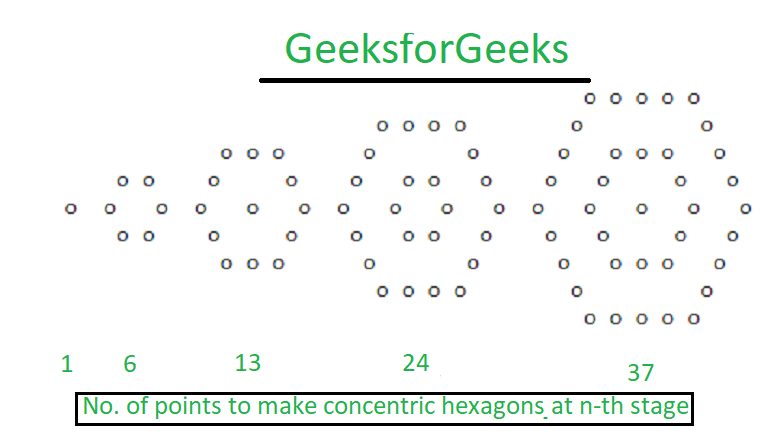# Concentric Hexagonal Numbers

The following numbers form the concentric hexagonal sequence :
0, 1, 6, 13, 24, 37, 54, 73, 96, 121, 150 ……

The number sequence forms a pattern with concentric hexagons, and the numbers denote the number of points required after the n-th stage of the pattern.Examples:

Input : N = 3
Output : 13

Input : N = 4
Output : 24

## Recommended: Please try your approach on {IDE} first, before moving on to the solution.

Approach :
The above series can be referred from Concentric Hexagonal Numbers.

Nth term of the series is 3*n2/2

Below is the implementation of the above approach :

## C++

 `// CPP program to find nth concentric hexagon number ` `#include ` `using` `namespace` `std; ` ` `  `// Function to find nth concentric hexagon number ` `int` `concentric_Hexagon(``int` `n) ` `{ ` `    ``return` `3 * ``pow``(n, 2) / 2; ` `} ` ` `  `// Driver code ` `int` `main() ` `{ ` `    ``int` `n = 3; ` ` `  `    ``// Function call ` `    ``cout << concentric_Hexagon(n); ` ` `  `    ``return` `0; ` `} `

## Java

 `// Java program to find ` `// nth concentric hexagon number ` `class` `GFG ` `{ ` `     `  `    ``// Function to find ` `    ``// nth concentric hexagon number ` `    ``static` `int` `concentric_Haxagon(``int` `n)  ` `    ``{ ` `        ``return` `3` `* (``int``)Math.pow(n, ``2``) / ``2``; ` `    ``} ` `     `  `    ``// Driver Code ` `    ``public` `static` `void` `main (String[] args) ` `    ``{ ` `        ``int` `n = ``3``; ` `         `  `        ``// Function call ` `        ``System.out.println(concentric_Haxagon(n)); ` `    ``} ` `} ` ` `  `// This code is contributed by ` `// sanjeev2552 `

## Python3

 `# Python3 program to find ` `# nth concentric hexagon number ` `  `  `# Function to find  ` `# nth concentric hexagon number ` `def` `concentric_Hexagon(n): ` ` `  `    ``return` `3` `*` `pow``(n, ``2``) ``/``/` `2` ` `  `# Driver code ` `n ``=` `3` ` `  `# Function call ` `print``(concentric_Hexagon(n)) ` `  `  `# This code is contributed by Mohit Kumar `

## C#

 `// C# program to find nth concentric hexagon number ` `using` `System; ` `class` `GFG ` `{ ` ` `  `// Function to find nth concentric hexagon number ` `static` `int` `concentric_Hexagon(``int` `n) ` `{ ` `    ``return` `3 * (``int``)Math.Pow(n, 2) / 2; ` `} ` ` `  `// Driver code ` `public` `static` `void` `Main() ` `{ ` `    ``int` `n = 3; ` ` `  `    ``// Function call ` `    ``Console.WriteLine(concentric_Hexagon(n)); ` `} ` `} ` ` `  `// This code is contributed by Nidhi `

Output:

```13
```

My Personal Notes arrow_drop_upCheck out this Author's contributed articles.

If you like GeeksforGeeks and would like to contribute, you can also write an article using contribute.geeksforgeeks.org or mail your article to contribute@geeksforgeeks.org. See your article appearing on the GeeksforGeeks main page and help other Geeks.

Please Improve this article if you find anything incorrect by clicking on the "Improve Article" button below.